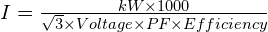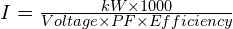# Calculate current of any Induction Motor in just 2 steps!

Calculate current of any Induction Motor in just 2 steps! 1024 576 Gaurav J

In this tutorial, I’ll explain the 2 simple steps to calculate current of any induction motor. Induction motor can be single phase or three phase. So, let’s get started.

## Step 1

##### Get the following data
• Rating of the motor in kW
• Operating Voltage
• Power Factor
• Efficiency of the motor

You’ll get all the above data on nameplate of the motor.

If power factor is not known you can assume it as Unity.

## Step 2

##### Put the values in following formula

For 3 phase motorsFor 1 phase motorsYou can put the values obtained in step 1 in relevant formula given above and calculate the current of induction motor!

Find out, how you can calculate Short Circuit current of any transformer is just 3 steps, click here.###### Gaurav J

Electrical Engineer. Content Creator. Currently working with a High & Extra High Voltage Switchgear Industry.

All stories by: Gaurav J